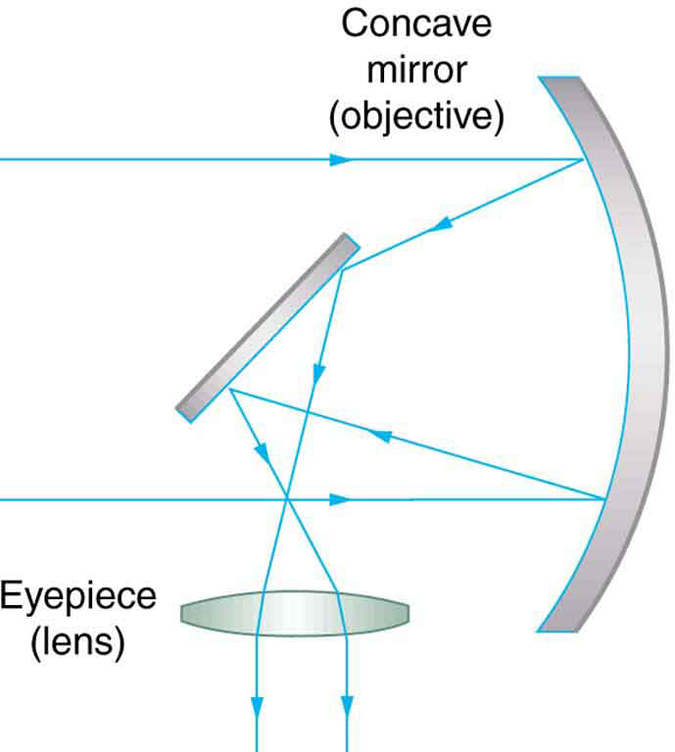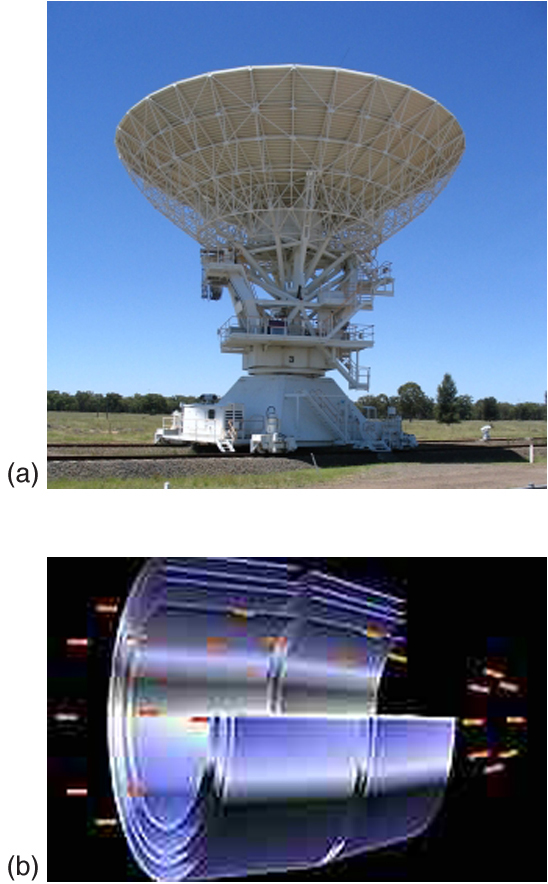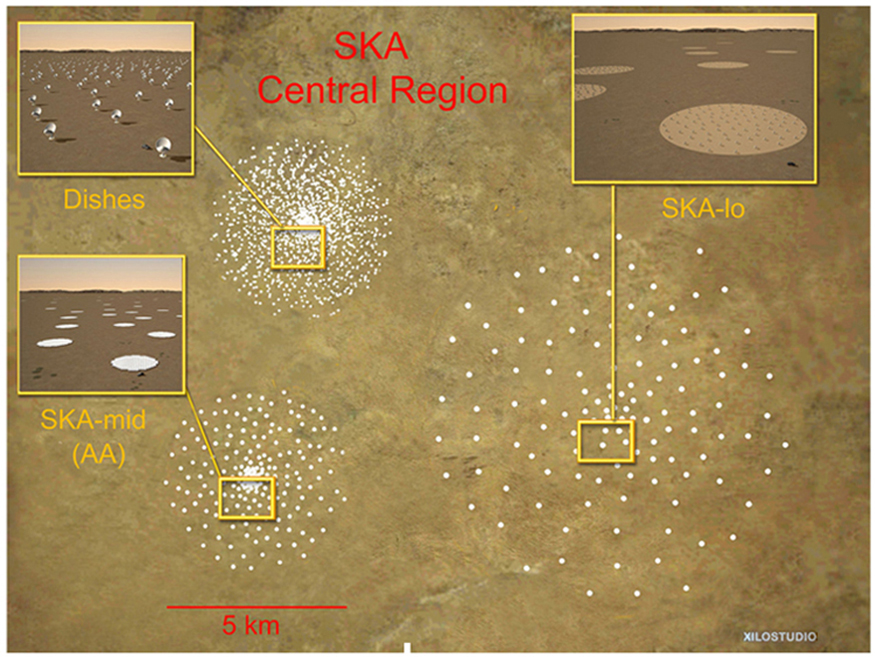# 26.5 Telescopes  (Page 2/4)

 Page 2 / 4

The image in most telescopes is inverted, which is unimportant for observing the stars but a real problem for other applications, such as telescopes on ships or telescopic gun sights. If an upright image is needed, Galileo’s arrangement in [link] (a) can be used. But a more common arrangement is to use a third convex lens as an eyepiece, increasing the distance between the first two and inverting the image once again as seen in [link] .This arrangement of three lenses in a telescope produces an upright final image. The first two lenses are far enough apart that the second lens inverts the image of the first one more time. The third lens acts as a magnifier and keeps the image upright and in a location that is easy to view.

A telescope can also be made with a concave mirror as its first element or objective, since a concave mirror acts like a convex lens as seen in [link] . Flat mirrors are often employed in optical instruments to make them more compact or to send light to cameras and other sensing devices. There are many advantages to using mirrors rather than lenses for telescope objectives. Mirrors can be constructed much larger than lenses and can, thus, gather large amounts of light, as needed to view distant galaxies, for example. Large and relatively flat mirrors have very long focal lengths, so that great angular magnification is possible.A two-element telescope composed of a mirror as the objective and a lens for the eyepiece is shown. This telescope forms an image in the same manner as the two-convex-lens telescope already discussed, but it does not suffer from chromatic aberrations. Such telescopes can gather more light, since larger mirrors than lenses can be constructed.

Telescopes, like microscopes, can utilize a range of frequencies from the electromagnetic spectrum. [link] (a) shows the Australia Telescope Compact Array, which uses six 22-m antennas for mapping the southern skies using radio waves. [link] (b) shows the focusing of x rays on the Chandra X-ray Observatory—a satellite orbiting earth since 1999 and looking at high temperature events as exploding stars, quasars, and black holes. X rays, with much more energy and shorter wavelengths than RF and light, are mainly absorbed and not reflected when incident perpendicular to the medium. But they can be reflected when incident at small glancing angles, much like a rock will skip on a lake if thrown at a small angle. The mirrors for the Chandra consist of a long barrelled pathway and 4 pairs of mirrors to focus the rays at a point 10 meters away from the entrance. The mirrors are extremely smooth and consist of a glass ceramic base with a thin coating of metal (iridium). Four pairs of precision manufactured mirrors are exquisitely shaped and aligned so that x rays ricochet off the mirrors like bullets off a wall, focusing on a spot.(a) The Australia Telescope Compact Array at Narrabri (500 km NW of Sydney). (credit: Ian Bailey) (b) The focusing of x rays on the Chandra Observatory, a satellite orbiting earth. X rays ricochet off 4 pairs of mirrors forming a barrelled pathway leading to the focus point. (credit: NASA)

A current exciting development is a collaborative effort involving 17 countries to construct a Square Kilometre Array (SKA) of telescopes capable of covering from 80 MHz to 2 GHz. The initial stage of the project is the construction of the Australian Square Kilometre Array Pathfinder in Western Australia (see [link] ). The project will use cutting-edge technologies such as adaptive optics    in which the lens or mirror is constructed from lots of carefully aligned tiny lenses and mirrors that can be manipulated using computers. A range of rapidly changing distortions can be minimized by deforming or tilting the tiny lenses and mirrors. The use of adaptive optics in vision correction is a current area of research.An artist’s impression of the Australian Square Kilometre Array Pathfinder in Western Australia is displayed. (credit: SPDO, XILOSTUDIOS)

## Section summary

• Simple telescopes can be made with two lenses. They are used for viewing objects at large distances and utilize the entire range of the electromagnetic spectrum.
• The angular magnification M for a telescope is given by
$M=\frac{\theta \prime }{\theta }=-\frac{{f}_{\text{o}}}{{f}_{\text{e}}}\text{,}$
where $\theta$ is the angle subtended by an object viewed by the unaided eye, $\theta \prime$ is the angle subtended by a magnified image, and ${f}_{\text{o}}$ and ${f}_{\text{e}}$ are the focal lengths of the objective and the eyepiece.

## Conceptual questions

If you want your microscope or telescope to project a real image onto a screen, how would you change the placement of the eyepiece relative to the objective?

## Problem exercises

Unless otherwise stated, the lens-to-retina distance is 2.00 cm.

What is the angular magnification of a telescope that has a 100 cm focal length objective and a 2.50 cm focal length eyepiece?

$-\text{40}\text{.}0$

Find the distance between the objective and eyepiece lenses in the telescope in the above problem needed to produce a final image very far from the observer, where vision is most relaxed. Note that a telescope is normally used to view very distant objects.

A large reflecting telescope has an objective mirror with a $\text{10}\text{.}0 m$ radius of curvature. What angular magnification does it produce when a $3\text{.}\text{00 m}$ focal length eyepiece is used?

$-1\text{.}\text{67}$

A small telescope has a concave mirror with a 2.00 m radius of curvature for its objective. Its eyepiece is a 4.00 cm focal length lens. (a) What is the telescope’s angular magnification? (b) What angle is subtended by a 25,000 km diameter sunspot? (c) What is the angle of its telescopic image?

A $7.5×$ binocular produces an angular magnification of $-7\text{.}\text{50}$ , acting like a telescope. (Mirrors are used to make the image upright.) If the binoculars have objective lenses with a 75.0 cm focal length, what is the focal length of the eyepiece lenses?

$+\text{10.0 cm}$

Consider a telescope of the type used by Galileo, having a convex objective and a concave eyepiece as illustrated in [link] (a). Construct a problem in which you calculate the location and size of the image produced. Among the things to be considered are the focal lengths of the lenses and their relative placements as well as the size and location of the object. Verify that the angular magnification is greater than one. That is, the angle subtended at the eye by the image is greater than the angle subtended by the object.

full meaning of GPS system
how to prove that Newton's law of universal gravitation F = GmM ______ R²
sir dose it apply to the human system
prove that the centrimental force Fc= M1V² _________ r
prove that centripetal force Fc = MV² ______ r
Kaka
how lesers can transmit information
griffts bridge derivative
below me
please explain; when a glass rod is rubbed with silk, it becomes positive and the silk becomes negative- yet both attracts dust. does dust have third types of charge that is attracted to both positive and negative
what is a conductor
Timothy
hello
Timothy
below me
why below you
Timothy
no....I said below me ...... nothing below .....ok?
dust particles contains both positive and negative charge particles
Mbutene
corona charge can verify
Stephen
when pressure increases the temperature remain what?
remains the temperature
betuel
what is frequency
define precision briefly
CT scanners do not detect details smaller than about 0.5 mm. Is this limitation due to the wavelength of x rays? Explain.
hope this helps
what's critical angle
The Critical Angle Derivation So the critical angle is defined as the angle of incidence that provides an angle of refraction of 90-degrees. Make particular note that the critical angle is an angle of incidence value. For the water-air boundary, the critical angle is 48.6-degrees.
okay whatever
Chidalu
pls who can give the definition of relative density?
Temiloluwa
the ratio of the density of a substance to the density of a standard, usually water for a liquid or solid, and air for a gas.
Chidalu
What is momentum
mass ×velocity
Chidalu
it is the product of mass ×velocity of an object
Chidalu
how do I highlight a sentence]p? I select the sentence but get options like copy or web search but no highlight. tks. src
then you can edit your work anyway you want
Wat is the relationship between Instataneous velocity
Instantaneous velocity is defined as the rate of change of position for a time interval which is almost equal to zero
AstronomyByBy Richley CrapoBy OpenStaxBy JavaChamp TeamBy Janet ForresterBy Subramanian DivyaBy OpenStaxBy Richley CrapoBy Richley CrapoBy OpenStaxBy OpenStax